#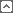###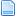Gönderen Konu: 5 x 3 = 5 + 5 + 5 ( teorik olarak yanlış)  (Okunma sayısı 1267 defa)

0 Üye ve 1 Ziyaretçi konuyu incelemekte.

Ocak 10, 2016, 07:18:50 ÖS
• Seyirci
• Orta Dereceli Uye
•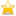• İleti: 222
• Cinsiyet: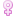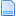##### 5 x 3 = 5 + 5 + 5 ( teorik olarak yanlış)
matrislerde ve fizikte ( kitaplar.ankara.edu.tr/dosyalar/pdf/734.pdf ) geçen bir hayli ilginç durum.makaleyi aynen paylaşıyorum.

kaynak site:  https://medium.com/i-math/why-5-x-3-5-5-5-was-marked-wrong-b34607a5b74c#.kinlvv84c

Why Was 5 x 3 = 5 + 5 + 5 Marked Wrong
Viral Math Problem Explained

A lot of you have been asking about WHY this viral math problem is marked incorrect. And thats a great question! It seems a little absurd, doesnt it?
Cloakenn/Imgur
Equals Versus Equivalency

Just because two things are equal doesnt mean theyre equivalent.

Equal is defined as, being the same in quantity, size, degree, or value. Whereas equivalent is defined as, equal in value, amount, function, or meaning. In the above problem 5 x 3 is equal to 5 + 5 + 5, but theyre not necessarily equivalent. Equivalence relates to meaning, so it depends on the meaning of multiplication.
I did something today Ive never done before,
I looked up the definition of multiplication.

To be able to apply the commutative property, we must first establish a definition of multiplication that applies meaning to the operand order. If operand order isnt important, the commutative property serves no useful distinction.

In Wikipedias definition of multiplication, they indicate the first factor is the number of copies and the second is the number being repeated.
Multiplication, Wikipedia

If this is the definition the teacher taught, 5 x 3 is equivalent to 5 copies of 3, or 3 + 3 + 3 + 3 + 3. It is equal but not equivalent to 5 + 5 + 5 because 3 copies of 5 represents something different.

For example, 3 bundles of 5 bananas is different from 5 bundles of 3 bananas although they total to the same number of bananas. Their structures are different.

Heres another example: 30 ÷ 2 is equal to 15. But does 30 ÷ 2 represent multiplication? Is it equivalent to repeated addition?

No, it represents division. It is equal to 5 times 3 but not equivalent.
I get the difference, but isnt it a little harsh?

It depends. If the teacher has already taught the commutative property of multiplication (the law that says a x b = b x a), then this is a fine substitution to make. And it is awesome that the student realized this! Kudos! What a mathlete!

If the teacher has not covered the commutative property, then it might be unwise to let a student continue with this line of thought if they dont understand the reasons why fully.

Its common for beginners to get confused as to when it is okay to switch the order of values in binary operations. We know that the following are not equal.

But this is easily confused with the child who sees that sometimes its okay to switch the order and other times its not and hasnt learned when or why.

By focusing on the meaning of these operations as they relate to repeated addition, arrays and area, teachers are creating a deeper understanding and trying to prevent students from making these kinds of mistakes.
Why does it matter if the meanings are slightly different?

Its more important than ever for students to understand the difference between equal as a result and equivalence in meaning from a young age because it is a fundamental computer science concept.

In programming, there is a distinction between testing if two things are equal or equivalent (aka identical).

Equal means they have the same end value, like 5 + 5 + 5 = 3  5 = 5  3 = 15. Equivalent means not only are they equal, they are also of the same data type. In other words, they mean the same thing.

Depending on the language, numbers and expressions that look the same dont always mean the same.

For example, in JavaScript if we test for equality with the == operator:

4 == 4 returns True

because the compiler understands both are referring to the number 4. But if we test for identity using the === operator:

4 === 4 returns False

because they mean different things. The first is a string whereas the second is a number, therefore they are not the same. This is just one example of how equality isnt always straightforward.

(Note: for a more in depth discussion on == vs === in JS, check out this stack overflow discussion.)
The Right Mindset for Matrix Multiplication

Notice that the second problem is marked incorrect as well. Why is it important that 4 x 6 is 4 rows of 6, instead of 6 rows of 4?

Not only does this adhere to the definition, it also teaches students the correct order for diagramming matrices, which is rows times columns.

Keeping rows and columns straight in matrix multiplication is vital.

Matrices are labeled using a row by column notation, m x n. To multiply matrices together, you multiply the rows of the first matrix by the columns of the second. The number of columns in the first matrix must equal the number of rows in the second, or else you cannot multiply them together.

For example, we can multiply a 2 x 3 matrix and a 3 x 4 matrix together. But if we swap the order there will not be sufficient rows and columns, and the operation cannot be performed.

Order is essential in the definition of multiplication because not all forms of multiplication are commutative, such as matrix multiplication. This is why it is taught as a separate property.
I know its frustrating but
Respect the Teachers

They are qualified experts on child education. They have the best intentions for the students in mind. This teacher made a decision based off a lot more information about the student and class setting than we can tell from a photo. We dont have to agree with it, but we can respect it. If you are confused, ask them why they did something before you discredit a teacher on the internet.
kalite çöplükte aranmaz.

Ekim 19, 2016, 08:42:37 ÖÖ
Yanıtla #1
• Orta Dereceli Uye
•• İleti: 291
• Cinsiyet: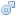##### Ynt: 5 x 3 = 5 + 5 + 5 ( teorik olarak yanlış)
Merhaba,

Öncelikle konu Türkçe olarak anlatılsa daha faydalı olurdu diye düşünüyorum.

Kendi yorumuma gelince, eşitlik olmama nedeni şu: eşitliğin her iki tarafı da 15 olarak çıktı (output) vermesine rağmen girdiler(input) aynı değil. yanlış denmesinin nedeni bu olsa gerek.

Saygılarımla
"İsteyen yavaş gitsin, ister hızlı koşsun, arayan bulur. İki elinle isteğe sarıl; çünkü istektir iyi yola kılavuz olan."
Mevlana

### Benzer Konular

Konu / Başlatan Yanıt Son Gönderilen:0 Yanıt
1848 Gösterim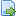Temmuz 31, 2007, 12:23:17 ÖÖ
Gönderen: shemuel3 Yanıt
3766 GösterimMayıs 12, 2009, 06:21:00 ÖS
Gönderen: Prenses Isabella9 Yanıt
5839 GösterimAğustos 12, 2019, 10:53:50 ÖS
Gönderen: karahan9 Yanıt
5699 GösterimTemmuz 06, 2013, 10:21:15 ÖS
Gönderen: ruzber0 Yanıt
1459 GösterimTemmuz 06, 2013, 06:48:07 ÖS
Gönderen: GOASISG8 Yanıt
3433 GösterimKasım 27, 2014, 06:44:43 ÖS6 Yanıt
6955 GösterimŞubat 13, 2017, 10:54:28 ÖS
Gönderen: Ankara0 Yanıt
1104 GösterimŞubat 04, 2015, 05:23:37 ÖÖ
Gönderen: Risus1 Yanıt
1179 GösterimŞubat 04, 2015, 11:50:26 ÖS
Gönderen: Waldow7 Yanıt
2059 GösterimNisan 09, 2015, 12:44:16 ÖS
Gönderen: Selim